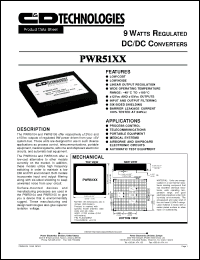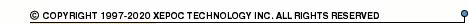More than4 307 195 components listedPWR51 series datasheets. Manufacturer: CANDD.

 PWR5104 9 watt regulated DC/DC converter. Nom.input voltage (typ.) 5VDC, rated output voltage +-12VDC, rated output current (max) +-375mA. in 5-pin - package. Operational temperature range from -25°C to 85°C. Datasheet*) PWR5104H 9 watt regulated DC/DC converter. Nom.input voltage (typ.) 5VDC, rated output voltage +-12VDC, rated output current (max) +-375mA. in 5-pin - package. Operational temperature range from -25°C to 85°C. Datasheet*) PWR5105 9 watt regulated DC/DC converter. Nom.input voltage (typ.) 5VDC, rated output voltage +-15VDC, rated output current (max) +-300mA. in 5-pin - package. Operational temperature range from -25°C to 85°C. Datasheet*) PWR5105H 9 watt regulated DC/DC converter. Nom.input voltage (typ.) 5VDC, rated output voltage +-15VDC, rated output current (max) +-300mA. in 5-pin - package. Operational temperature range from -25°C to 85°C. Datasheet*) PWR51XX 9 WATT REGULATED DC/DC CONVERTER Datasheet*)